# Database test questions for interview, exams, entrance

## Database test questions for interview, exams, entrance

(1) Data items P and Q in the following transaction are initialized to zero:

If P = 0 then Q: = Q+1;
Write (Q);

If Q = 0 then P: = P+1;

Write (P);

Any non-serial interleaving of T1 and T2 for concurrent execution leads to

(A) A schedule that is not conflict serializable
(B) A schedule for which a precedence graph cannot be drawn
(C) A serializable schedule
(D) A conflict serializable schedule

ANSWER: A schedule that is not conflict serializable

(2) A bank account has initial balance of 12000 and a mortgage payment of 2000 is transferred and then 5% interest is applied. T1 and T2 are the two transactions whose log sequence on bank account is given below: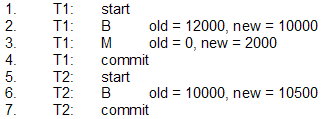Before log record 7 is written the database system is crashed. Which one statement is true of the recovery procedure when the system is restarted?

(A) We can apply redo and undo operations in arbitrary order
(B) We must redo log record 6 to set B to 10500
(C) We must undo log record 6 to set B to 10000 and then redo log records 2 and 3
(D) We need not redo log records 2 and 3

ANSWER: We need not redo log records 2 and 3

(3) The two relation schemes R1 (A, B) and R2 (C, D) has two corresponding relation instances r1 and r2. B from R1 is the foreign key that refers to C in R2. The statement that always holds true if data in r1 and r2 satisfy referential integrity constraints is

(A) πB (r1) – πC (r2) ≠E
(B) πB (r1) = πC (r2)
(C) πB (r1) – πC (r2) = E
(D) πC (r2) – πB (r1) = E

ANSWER: πB (r1) – πC (r2) = E

(4) The relation R that has x number of tuples is joined with relation S that has y number of tuples. The maximum and minimum sizes of join respectively are

(A) xy and x + y
(B) x + y and I x - yI
(C) xy and 0
(D) x + y and 0

(5) Consider the relation book (title, price) that contain title and price of different books. No two books have same price. What will the SQL query given below list?
Select title from book as B where (select count (*) from book as T where T.price>B.price)<5

(A) Titles of the five most expensive books
(B) Titles of the fifth most expensive books
(C) Titles of the fifth most inexpensive books
(D) Titles of the four most expensive books

ANSWER: Titles of the five most expensive books

For questions 6 and 7 refer to the data given below: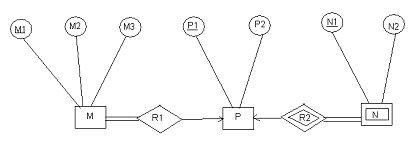(6) To represent M, N, P, R1, R2 the minimum number of tables needed is

(A) 3
(B) 6
(C) 4
(D) 2

(7) For the correct answer to the above question a correct attribute set for one of the tables is

(A) {M1, P1, N1}
(B) {M1, M2, M3, P1}
(C) {M1, M2, P1}
(D) {M1, P1}

(8) Consider a B+ tree in which order of the internal node is 3 and that of the leaf node is 2. The key values are inserted into this tree in the order given as 10, 3, 6, 8, 4, 2, 1. The order of the internal node is the maximum number of tree pointers in each node and the order of the leaf node is the maximum number of data items that can be stored in it. Assume that the B+ tree is initially empty. As a result of this iteration the maximum number of times leaf nodes would get split up is

(A) 2
(B) 3
(C) 4
(D) 5

(9) Consider a file with following specifications:

File has 16384 records
Each record is 32 byte long
Size of the key field is 6byte
Organization of file is unspanned
File is ordered in on a non-key field
File is stored in a file system with block size 1024byte
Size of the block pointer is 10 byte
Secondary index is built on the key field of file
Multi-level index scheme is used to store the secondary index.

The number of first level and second level blocks in the multi-level index is respectively

(A) 512 and 4
(B) 256 and 4
(C) 128 and 8
(D) 8 and 0

(10) Consider (x, y) ∈ geq only if y ≥ x where geq is the relation that represents “greater than or equal to”.

Create table geq
(lb integer not null
ub integer not null
primary key lb
foreign key (ub) references geq on delete cascade)

If the tuple (x, y) is deleted

(A) The deletion of (x, y) is prohibited
(B) A tuple (z, w) with z>y is deleted
(C) A tuple (z, w) with z>x is deleted
(D) A tuple (z, w) with w

ANSWER: A tuple (z, w) with w

(11) Which one of the following statement is incorrect for the basic ER and relational models?

(A) An attribute of an entity can be composite
(B) An attribute of an entity can have more than one value
(C) An attribute can have exactly one value or a NULL value in a row of a relational table.
(D) An attribute can have more than one value in a row of a relational table.

ANSWER: An attribute can have more than one value in a row of a relational table.

(12) We have two relations R (a, b, c) and S (d, e, f) in which d is the foreign key of S that refers to the primary key of R. The operations on R and S are

a. Insert into R
b. Insert into S
c. Delete from R
d. Delete from S

The statement that holds true about the referential integrity constraint above is

(A) b and c can cause its violation
(B) a and d can cause its violation
(C) a, b and d can cause its violation
(D) a, b, c and d can cause its violation

ANSWER: b and c can cause its violation

For questions 13 and 14 refer to the data given below:

We have the relations A, B and C given below: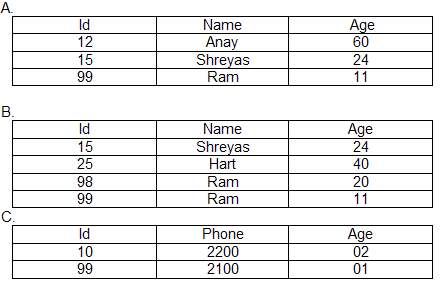(13) Assuming that the schema of A E B is the same as that of A the number of tuples the result of relational algebraic expression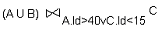contain is

(A) 7
(B) 3
(C) 5
(D) 8

(14) Consider an SQL query

SELECT A.Id FROM A WHERE A.Age>
All (SELECT B.Age FROM B WHERE B.name = ‘Anay’)

The result of this query contains

(A) 1 tuple
(B) 2 tuples
(C) 3 tuples
(D) 4 tuples

(15) Which one of the following statement about B+ trees is correct?

(A) B+ trees are for secondary indexes and B trees are for primary indexes
(B) B+ trees are for main memory and B trees are for storing data on disk
(C) B+ trees height is independent of the number of records
(D) On B+ trees range queries are faster

ANSWER: On B+ trees range queries are faster

(16) Consider the database given below: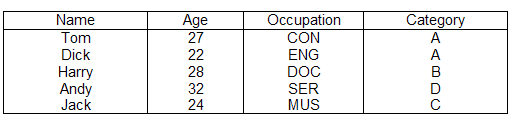For the above table the index file associated contains the values 1, 3, 2, 5 and 4. Index is built from the field

(A) Occupation
(B) Age
(C) Name
(D) Category

(17) Relation R and relation S are decomposed using set of functional dependencies F and G respectively. One decomposition is definitely BCNF and the other one is definitely 3NF but it is not known exactly which is which. In order to make the guaranteed identification the tests that should be done on decomposition is

(A) Lossless join
(B) BCNF definition
(C) 3NF definition
(D) Dependency preservation

(18) Which one of the following statement about SQL is correct?

(A) SQL permits attribute names to be repeated in the same relation
(B) If there are no indexes on the relations then an SQL query will not work
(C) Duplicates are automatically eliminated by an SQL query
(D) None of the above

For questions 19 and 20 refer to the data given below:

The relation R has eight attributes ABCDEFGH. For this relation the set of functional dependencies is F = {CH->G, A->BC, B->CFH, E->A, F->EG}. Field of R contains only atomic values. The set of F is exactly the set of FD’s that hold for R

(19) The number of candidate keys the relation R has is

(A) 7
(B) 2
(C) 4
(D) 6

(20) The relation R is

(A) In BCNF
(B) In 3NF but not in 4NF
(C) In 2NF but not in 3NF
(D) In 1NF but not in 2NF

ANSWER: In 1NF but not in 2NF

(21) The dependencies for the schema R (S T U V) are S->T, T->U, U->V and V->S. The decomposition R = (R1 and R2) is such that R1∩R2 = Φ. Which of the following statement holds true?

(A) The decomposition is both in 3NFand 2NF
(B) The decomposition is in 3NF but not in 2NF
(C) The decomposition is in 2NF but not in 3NF
(D) The decomposition is in 3NF

ANSWER: The decomposition is in 3NF but not in 2NF

(22) For normal relational database design the form that is considered adequate is

(A) 3NF
(B) 5NF
(C) 2NF
(D) 4NF

(23) The instance of the relational schema R (M, N, Q) is given below: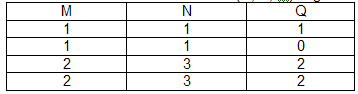We can conclude that

(A) M does not functionally determines N and N does not functionally determines Q
(B) N does not functionally determines Q
(C) M functionally determines N and N functionally determines Q
(D) M functionally determines N and N does not functionally determines Q

ANSWER: M functionally determines N and N does not functionally determines Q

(24) The relations in SQL can contain null values and comparisons with null values are treated as unknown. If all comparisons with null values are treated as false then the pair that is not equivalent is

(A) x=5 x>4 and x<6, where x is an integer
(B) x≠5 not (x=5)
(C) x=5 not (not (x=5))
(D) None of the above

(25) The functional dependencies for the relational schema student performance (name, courseNo, rollno, grade) is given below:

name -> rollno
rollno-> name

What is the highest normal form of this relational schema?

(A) 3NF
(B) 4NF
(C) BCNF
(D) 2NF

(26) The functional dependencies that are satisfied by the relation instance given below are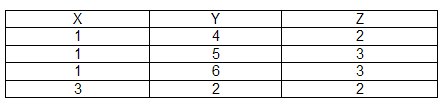(A) YZ->X and X->Z
(B) XY->Z and Z->Y
(C) YZ->X and Y->Z
(D) XZ->Y and Y->X

(27) For the relation R (A, B, C, D) the option that does not have a lossless join, dependency preserving BCNF decomposition is

(A) A->BCD
(B) A->B, B->C, C->D
(C) A->B, B->CD

(28) The maximum possible size of the file in the index allocation scheme of blocks to a file depends on

(A) The size of the blocks and the size of the address of the blocks
(B) The number of blocks used for the index, the size of the blocks and the size of the address of the blocks
(C) The number of blocks used for the index and the size of the blocks
(D) None of the above

ANSWER: The number of blocks used for the index and the size of the blocks

(29) Consider the relation’s r (w, x) and s (y, z).

select distinct w, x from r, s. The result of this select statement is guaranteed to be same as r provided

(A) s has no duplicates and r is non-empty
(B) r has no duplicates and s is non-empty
(C) r and s have no duplicates
(D) None of the above

ANSWER: r has no duplicates and s is non-empty

(30) Which of the following option is false if we have the functional dependencies given below?

AB->CD, AF->D, DE->F, C->G, F->E, G->A

(A) {AF+ } = {ACDEFG}
(B) {CF+ } = {ACDEFG}
(C) {BG+ } = {ACDFG}
(D) {AB+ } = {ABCDFG}

(31) For the relational schema R = (A, B, C, D, E, H) the functional dependencies that hold is {A->B, BC->D, E->C, D->A}. The candidate keys of R is

(A) AEH, BEH, BCH
(B) AE, BE
(C) AEH, BEH, DEH
(D) AE, BE, DE

(32) We have the table given below: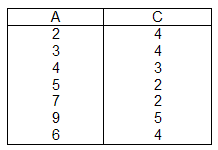The two attributes are A and C where A is the primary key and C is the foreign key referencing a with on-delete cascade. When the tuple (2, 4) is deleted, to preserve referential integrity the set of all tuples that must be additionally deleted is

(A) (3, 4) and (6, 4)
(B) (5, 2) and (7, 2)
(C) (3, 4), (4, 3) and (6, 4)
(D) (5, 2), (7, 2) and (9, 5)

ANSWER: (5, 2), (7, 2) and (9, 5)

(33) The order of an internal node in a B+ tree index is the maximum number of children it can have. If 6byte is occupied by a child pointer, 14 bytes by the search field value and 512 byte by the block size then the order of internal node is

(A) 23
(B) 24
(C) 25
(D) 26

(34) In an E/R diagram E1 and E2 are the two entities with single value attributes. Relationship between E1 and E2 are represented by R1 and R2 where R1 is one-to-many and R2 is many-to-many. The minimum number of tables required to represent this situation in the relational model is

(A) 8
(B) 3
(C) 1
(D) 2

(35) In the table given below T1, T2 and T3 are the transactions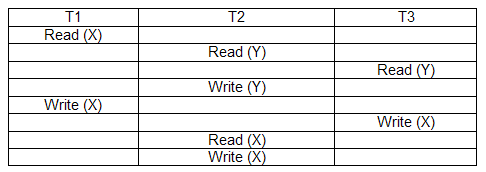The correct serialization of the above schedules is

(A) T2->T3->T1
(B) T3->T2->T1
(C) T1->T2->T3
(D) T1->T3->T2

(36) The statement that does not hold true is

(A) A prime attribute can be transitively dependent on a key in a 3NF relation
(B) In 2NF every key has only one attribute
(C) A prime attribute can be transitively dependent on a key in a BCNF relation
(D) In BCNF any relation has two attributes

ANSWER: A prime attribute can be transitively dependent on a key in a BCNF relation

(37) The adjacency relation of vertices in a graph is represented in a table Adj (X, Y). The query that cannot be expressed by a relational algebra expression of constant length is

(A) List of all vertices, which belong to cycles of less than three vertices
(B) List of all vertices, which have self loops
(C) List of all vertices adjacent to a given vertex
(D) List of all vertices reachable from a given vertex

ANSWER: List of all vertices, which belong to cycles of less than three vertices

(38) In a B+ tree the order of a leaf node is defined as the maximum number of (value, data record pointer) pairs it can hold. Block size = 1kbyte, data record pointer = 7 byte long, value field = 9byte long, block pointer = 6byte long. The order of the leaf node is

(A) 64
(B) 46
(C) 76
(D) 67

(39)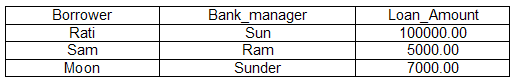The SQL Query for the database table, which is given by the name, Loan is given below.
SELECT count (*)
FROM ((SELECT Borrower. Bank_manager FROM Loan) AS S NATURAL JOIN (SELECT Bank_manager, Loan_amount FROM Loan) AS T);

What is the output of this query?

(A) 6
(B) 3
(C) 8
(D) 2

Enroll (rollno, courseno, coursename)

The above statement is the relational schema pertaining to a student’s database where the primary keys are shown underlined. In the student table the number of tuples is 120 and in the Enroll tables the number of tuples is 8. In (student * Enroll) where * represents natural join the maximum and minimum number of tuples are

(A) 250, 35
(B) 900, 8
(C) 8, 8
(D) 120, 20

(41) We have a schema R (A, B, C, D) and functional dependencies A->B and C->D. Then the decomposition of R into R1 (AB) and R2 (CD) is

(A) Lossless join but not dependency preserving
(B) Dependency preserving and lossless join
(C) Not dependency preserving and not lossless join
(D) Dependency preserving but not lossless join

ANSWER: Dependency preserving but not lossless join

(42) Consider the relation R (A, B, C) that contain 200 tuples and the relation S (B, D, E) that contains 100 tuples. The functional dependencies shown below holds for relation R and S

B->A,
A->C

The maximum number of tuples possible in the natural join of R and S is

(A) 700
(B) 500
(C) 100
(D) 200

(43) Which one of the following statement is true with regard to the expressive power of the formal relational query languages?

(A) Relational algebra and safe relational calculus both have same power
(B) Relational algebra and relational calculus both have same power
(C) Relational algebra is more powerful than relational calculus
(D) None of the above

ANSWER: Relational algebra and safe relational calculus both have same power

(44) The key factor that is responsible for preferring B+ trees to binary search trees for indexing database relations is

(A) Database relations have a large number of records
(B) Data transfer from disks is in blocks
(C) B+ trees requires less memory than binary search trees
(D) Database relations are stored on the primary key

ANSWER: Data transfer from disks is in blocks

(45) Relation R with an associated set of functional dependencies F, is decomposed into BCNF. The redundancy (arising out of functional dependencies) in the resulting set of relations is

(A) Indeterminate
(B) Zero
(C) Proportional to size of F
(D) More than zero but less than that of an equivalent 3NF decomposition

(46) The statement that does not hold true about normal forms is

(A) Any relation with two attributes is in BCNF
(B) BCNF is stricter than 3NF
(C) Lossless, dependency-preserving decomposition into 3NF is always possible
(D) Lossless, dependency-preserving decomposition into BCNF is always possible

ANSWER: Lossless, dependency-preserving decomposition into BCNF is always possible

(47) Of the four scenario given below the one that may lead to an irrecoverable error in a database system is

(A) A transaction reads a data item after it is written by an uncommitted transaction
(B) A transaction reads a data item after it is written by a committed transaction
(C) A transaction reads a data item after it is read by an uncommitted transaction
(D) A transaction writes a data item after it is read by an uncommitted transaction

ANSWER: A transaction reads a data item after it is written by an uncommitted transaction

(48) Which type of clustering index is defined on the fields?

(A) Non-key and non-ordering
(B) Non-key and ordering
(C) Key and non-ordering
(D) Key and Ordering

(49) If the maximum number of keys in a node of a B+ tree is 5 then the minimum number of keys in any non-root node is

(A) 4
(B) 3
(C) 2
(D) 1

(50) The concurrency control protocol that ensure both conflict serializability and freedom from deadlock is

(A) Time stamp ordering
(B) 2-phase locking
(C) Both A and B
(D) Neither A nor B